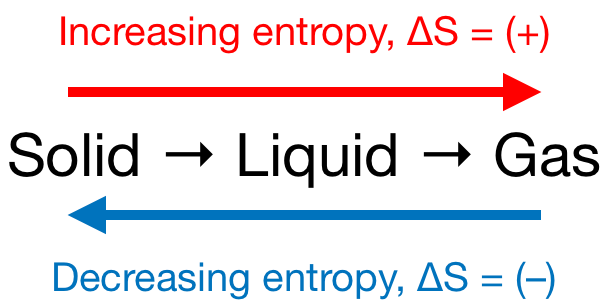# Problem: Predict how the entropy of the substance is affected in the following processes: a) Ar(l) → Ar(g)b) Cu(s, 300 K) → Cu(s, 800 K)c) H2O (g, 75 Torr, 300 K) → H2O(g, 150 Torr, 300 K)Which of the following reactions have a positive ΔSrxn? Check all that apply. a) A(s) + B(s) → C(g)b) A(g) + B(g) → C(g)c) 2A(g) + B(g) → 4C(g)d) 2A(g) + B(g) → C(g)

###### FREE Expert Solution

We’re being asked to predict how the entropy of each substance is affected by the given process

Recall that entropy is the degree of chaos or disorder of a system and that it depends on the following:

1. Phase: The gas phase has the highest entropy, followed by the liquid, and then the solid phase with the lowest entropy.

Gas > Liquid > Solid

2. Complexity: More elements in the compound leads to higher entropy.

Ex. NO3(g) [4 elements] has higher entropy than NO2(g) [3 elements].

3. Mass: The greater the mass, then the greater the entropy.

Ex. Xe(g) [greater mass] has higher entropy than He(g).

For a given process, entropy (ΔS) will either increase or decrease.

Remember that phase changes lead to a change in entropy:###### Problem Details

Predict how the entropy of the substance is affected in the following processes:

a) Ar(l) → Ar(g)

b) Cu(s, 300 K) → Cu(s, 800 K)

c) H2O (g, 75 Torr, 300 K) → H2O(g, 150 Torr, 300 K)

Which of the following reactions have a positive ΔSrxn? Check all that apply.

a) A(s) + B(s) → C(g)

b) A(g) + B(g) → C(g)

c) 2A(g) + B(g) → 4C(g)

d) 2A(g) + B(g) → C(g)